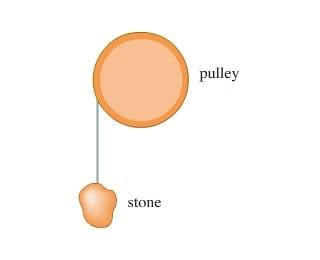# From rotational KE to translational displacement

## Homework Statement

A frictionless pulley has the shape of a uniform solid disk of mass 5.00 kg and radius 28.0 cm . A 1.40 kg stone is attached to a very light wire that is wrapped around the rim of the pulley, and the stone is released from rest. As it falls down, the wire unwinds without stretching or slipping, causing the pulley to rotate. How far must the stone fall so that the pulley has 3.10 J of kinetic energy?
Here's the given figure:## Homework Equations

Rotational KE = (1/2) Iω2
K1 + U1 = K2 + U2
ν = rω
a(tan)= dv/dt = r (dω/dt) = rα

## The Attempt at a Solution

Part A: Forces doing work on the system? Gravitational force
Part B:The magnitude of the velocity of the stone is the same as that of the point of contact.
Part C: How far must the stone fall so that the pulley has 3.10 J of kinetic energy?
I'm confused as to how I'm supposed to go from the above equations to finding linear displacement. Does this problem involve an integral of some kind?
Thanks so much!